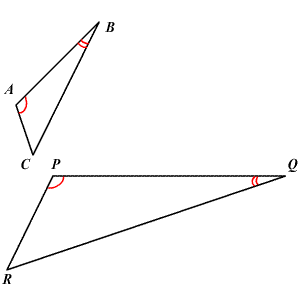# AA (Angle-Angle) Similarity

In two triangles, if two pairs of corresponding angles are congruent, then the triangles are similar .

(Note that if two pairs of corresponding angles are congruent, then it can be shown that all three pairs of corresponding angles are congruent, by the Angle Sum Theorem.)In the figure above, since $\angle A\cong \angle P$ and $\angle B\cong \angle Q$ , by AA similarity, $\Delta ABC\sim \Delta PQR$ .

Important Note:

Similar figures:

When figures have the same shape but may be different in size, they are called similar figures.

Congruent figures:

Figures that are the same size and the same shape are congruent figures.